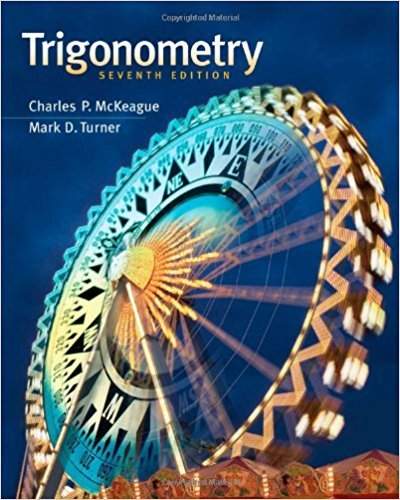×
×

Textbooks / Math / Trigonometry 7

# Trigonometry 7th Edition - Solutions by Chapter## Full solutions for Trigonometry | 7th Edition

ISBN: 9781111826857Trigonometry | 7th Edition - Solutions by Chapter

Solutions by Chapter
4 5 0 402 Reviews
##### ISBN: 9781111826857

This expansive textbook survival guide covers the following chapters: 85. This textbook survival guide was created for the textbook: Trigonometry, edition: 7. Trigonometry was written by and is associated to the ISBN: 9781111826857. The full step-by-step solution to problem in Trigonometry were answered by , our top Math solution expert on 12/27/17, 07:46PM. Since problems from 85 chapters in Trigonometry have been answered, more than 22455 students have viewed full step-by-step answer.

Key Math Terms and definitions covered in this textbook
• Block matrix.

A matrix can be partitioned into matrix blocks, by cuts between rows and/or between columns. Block multiplication ofAB is allowed if the block shapes permit.

• Column picture of Ax = b.

The vector b becomes a combination of the columns of A. The system is solvable only when b is in the column space C (A).

• Covariance matrix:E.

When random variables Xi have mean = average value = 0, their covariances "'£ ij are the averages of XiX j. With means Xi, the matrix :E = mean of (x - x) (x - x) T is positive (semi)definite; :E is diagonal if the Xi are independent.

• Cyclic shift

S. Permutation with S21 = 1, S32 = 1, ... , finally SIn = 1. Its eigenvalues are the nth roots e2lrik/n of 1; eigenvectors are columns of the Fourier matrix F.

• Diagonal matrix D.

dij = 0 if i #- j. Block-diagonal: zero outside square blocks Du.

• Exponential eAt = I + At + (At)2 12! + ...

has derivative AeAt; eAt u(O) solves u' = Au.

• Fast Fourier Transform (FFT).

A factorization of the Fourier matrix Fn into e = log2 n matrices Si times a permutation. Each Si needs only nl2 multiplications, so Fnx and Fn-1c can be computed with ne/2 multiplications. Revolutionary.

• Fourier matrix F.

Entries Fjk = e21Cijk/n give orthogonal columns FT F = nI. Then y = Fe is the (inverse) Discrete Fourier Transform Y j = L cke21Cijk/n.

• Full row rank r = m.

Independent rows, at least one solution to Ax = b, column space is all of Rm. Full rank means full column rank or full row rank.

• Hypercube matrix pl.

Row n + 1 counts corners, edges, faces, ... of a cube in Rn.

• Indefinite matrix.

A symmetric matrix with eigenvalues of both signs (+ and - ).

• Left inverse A+.

If A has full column rank n, then A+ = (AT A)-I AT has A+ A = In.

• Left nullspace N (AT).

Nullspace of AT = "left nullspace" of A because y T A = OT.

• Matrix multiplication AB.

The i, j entry of AB is (row i of A)·(column j of B) = L aikbkj. By columns: Column j of AB = A times column j of B. By rows: row i of A multiplies B. Columns times rows: AB = sum of (column k)(row k). All these equivalent definitions come from the rule that A B times x equals A times B x .

• Orthogonal subspaces.

Every v in V is orthogonal to every w in W.

• Row picture of Ax = b.

Each equation gives a plane in Rn; the planes intersect at x.

• Schur complement S, D - C A -} B.

Appears in block elimination on [~ g ].

• Singular matrix A.

A square matrix that has no inverse: det(A) = o.

• Sum V + W of subs paces.

Space of all (v in V) + (w in W). Direct sum: V n W = to}.

• Transpose matrix AT.

Entries AL = Ajj. AT is n by In, AT A is square, symmetric, positive semidefinite. The transposes of AB and A-I are BT AT and (AT)-I.

×